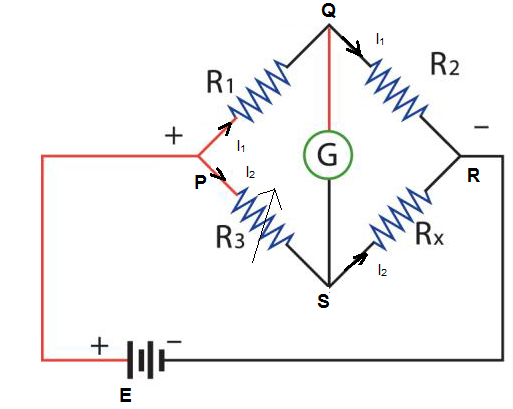# Wheatstone Bridge Formula

Wheatstone Bridge Circuit diagram is as shown in the picture belowR1 and R2 are known Resistances

Rx = Unknown Resistance

G = Galvanometer connected between Q and S

Battery connected between P and R terminals

I(1)= current that passes through R1 and R2

I(2) = current that passes through R3 and Rx

Considering the loop PSQP

$$\begin{array}{l}-I_{2}R_{3}+0+I_{1}R_{1}=0\end{array}$$

Considering the loop RQSR

$$\begin{array}{l}I_{1}R_{2}+0-I_{2}R_{x}=0\end{array}$$

From the first loop

$$\begin{array}{l}\frac{I_{2}}{I_{1}}=\frac{R_{1}}{R_{3}}\end{array}$$

From second loop

$$\begin{array}{l}\frac{I_{2}}{I_{1}}=\frac{R_{2}}{R_{x}}\end{array}$$

Hence to find the unknown resistance

$$\begin{array}{l}\frac{R_{1}}{R_{3}}=\frac{R_{2}}{R_{x}}\end{array}$$

$$\begin{array}{l}R_{x}=\frac{R_{2}R_{3}}{R_{1}}\end{array}$$